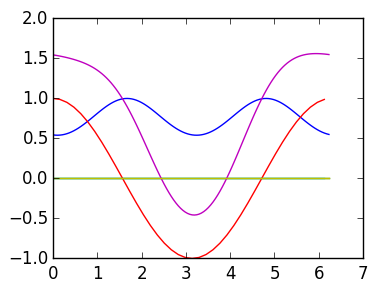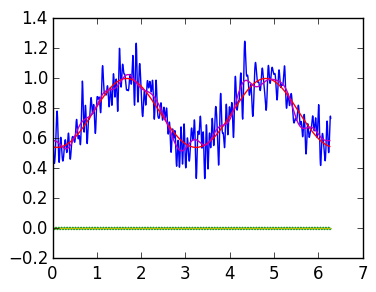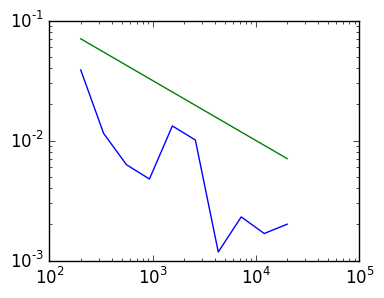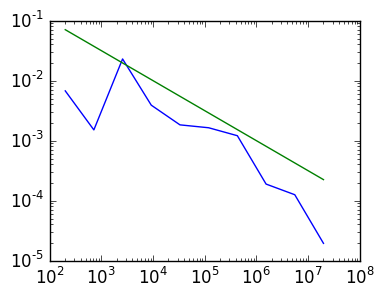# Assignment 3: The DFT, function approximation and signal smoothing¶

In this lecture we will create a type called FourFun to represent functions periodic on [0,2π). This will allow us to do the following:

n=11        # number of points used
f=FourFun(θ->cos(cos(θ-0.1)),n)  # calculate the Fourier coefficients of cos(cos(θ-0.1))
f(0.1)      # evaluate the approximate Fourier series at 0.1
plot(f)     # plots h


g=FourFun(θ->cos(θ),3)    # another function with a different number of coefficients
h=f+g       # add two Fourier series with different lengths
h(0.5)      # should approximate cos(cos(0.4)) + cos(0.5)


We will use this type to do signal smoothing:

f=FourFun(θ->cos(cos(θ-0.1)) + 0.1randn(),10000)
g=resize(f,-12,12)   # drops all but the coefficients between -12 and 12
f(0.5)   # should be approximately cos(cos(θ-0.1))


# FourFun type¶

The starting point is creating the type FourFun, that will represent the finite Fourier series $$\sum_{k=\alpha}^\beta f_k e^{i k \theta}$$

It has two fields: negative, containing the negative coefficients in decreasing order, that is, $[ f_{-1}, f_{-2}, \ldots, f_\alpha]$ and nonnegative, containing the non-negative coefficients in increasing order, that is, $[ f_0, f_1, \ldots, f_\beta]$.

In :
type FourFun
negative::Vector{Complex128}
nonnegative::Vector{Complex128}
end


Exercise 1(a) Complete the following function FourFun that calculates n Fourier coefficients of f, and returns FourFun. You can assume n is an integer, and if desired you can use the fft. (Hint: the fft assumes the values of the function are at $[\theta_0,\theta_1,\ldots,\theta_{n-1}]$ where $\theta_k = {2 \pi k \over n}$. Using the fft will save some time in the last exercise.)

In :
points(n) = linspace(0,2π-2π/n,n)

# INPUT: a Function and an Integer
# OUTPUT: a FourFun whose coefficients correspond to the Fourier series that interpolates
#  f at the points [θ_0,θ_1,…,θ_{n-1},θ_n]

function FourFun(f::Function,n::Integer)
p=points(n)
cfs=fft(map(f,p))/n
FourFun(cfs[end:-1:n÷2+2],cfs[1:n÷2+1])
end

Out:
FourFun

Exercise 1(b) Complete the following override of call to evaluate the finite Fourier series represented by f at the point t:

$$\sum_{k=\alpha}^\beta f e^{i k t}$$
In :
import Base.call

# INPUT: a FourFun f and a point 0≤t≤2π
# OUTPUT: The finite Fourier series that f encodes evalauted at t

function call(f::FourFun,θ)
ret=0.+0.im
for k=1:length(f.negative)
ret+=f.negative[k]*exp(-im*k*θ)
end
for k=1:length(f.nonnegative)
ret+=f.nonnegative[k]*exp(im*(k-1)*θ)
end
ret
end

Out:
call (generic function with 1089 methods)
In :
f=FourFun(θ->cos(cos(θ-0.1)),101)

f(0.2)-cos(cos(0.1))

Out:
-1.1102230246251565e-16 + 4.3007516003628927e-17im
In :
f=FourFun(θ->cos(cos(θ-0.1)),4)

Out:
FourFun(Complex{Float64}[-2.7755575615628914e-17 + 0.0im],Complex{Float64}[0.7697600889426757 + 0.0im,-2.7755575615628914e-17 + 0.0im,-0.22526069311488728 + 0.0im])

Exercise 1(c) Override + to sum two finite Fourier series. Make sure that you pad the lengths of the vectors! Test that the example at the beginning works.

In :
function padplus(a::Vector,b::Vector)
if length(a) ≥ length(b)
ret=copy(a)
ret[1:length(b)]+=b
ret
else
ret=copy(b)
ret[1:length(a)]+=a
ret
end
end

# INPUT: Two FourFuns f and g
# OUTPUT: A FourFun whose nonnegative/negative coefficients are the addition
#   of the nonnegative/negative coefficients of f and g.  Make sure you pad the
#   vectors to have the same length.

import Base.+
function +(f::FourFun,g::FourFun)
FourFun(neg,pos)
end

Out:
+ (generic function with 172 methods)
In :
g=FourFun(θ->cos(θ),3)    # another function with a different number of coefficients
h=f+g
g(0.1)+f(0.1) - h(0.1)

Out:
0.0 + 0.0im

# Plotting¶

Exercise 2(a) Override plot(f::FourFun) to plot both the real and imaginary parts of the finite Fourier series encoded by f. Make sure the number of plot points depends on the number of coefficients so that the function looks smooth regardless of the length. (Hint: it's faster to use ifft, but not mandatory.)

In :
using PyPlot

import PyPlot.plot

function plot(f::FourFun)
n=length(f.negative) + length(f.nonnegative)
p=points(10n+10)
vals=f(p)
plot(p,real(vals))
plot(p,imag(vals))
end

Out:
plot (generic function with 2 methods)

Exercise 2(b) Plot f, g and f+g where

f=FourFun(θ->cos(cos(θ-0.1)),11)
g=FourFun(θ->cos(θ),3)

In :
f=FourFun(θ->cos(cos(θ-0.1)),11)
g=FourFun(θ->cos(θ),3)

plot(f)
plot(g)
plot(f+g);## Signal smoothing¶

Exercise 3(a) Write a routine resize(f::FourFun,a,b) that resizes the Fourier coefficients to have -a negative coefficients and b+1 nonnegative coefficients. You can assume -a ≤ length(f.negative) and b +1 ≤ length(f.nonnegative).

In :
# INPUT: a FourFun f, a negative integer a and a positive integer b
# OUTPUT: a FourFun, with the first -a negative coefficients and
#         the first b+1 nonnegative coefficients of f

function resize(f::FourFun,α,β)
FourFun(f.negative[1:-α],f.nonnegative[1:β+1])
end

Out:
resize (generic function with 1 method)

Exercise 3(b) Plot the FourFun approximations of

f=FourFun(θ->cos(cos(θ-0.1)),25)

noisycos(θ::Number) = cos(cos(θ-0.1)) + 0.1randn()
noisycos(θ::Vector) = cos(cos(θ-0.1)) + 0.1randn(length(θ))

fr=FourFun(noisycos,200)
g=resize(fr,-12,12)

In :
f=FourFun(θ->cos(cos(θ-0.1)),25)

noisycos(θ::Number) = cos(cos(θ-0.1)) + 0.1randn()
noisycos(θ::Vector) = cos(cos(θ-0.1)) + 0.1randn(length(θ))

fr=FourFun(noisycos,200)
g=resize(fr,-12,12)

plot(fr)
plot(f)
plot(g)Out:
1-element Array{Any,1}:
PyObject <matplotlib.lines.Line2D object at 0x3119e7cd0>

Exercise 3(c) Use a loglog estimate the convergence rate of

fr=FourFun(θ->cos(cos(θ-0.1)) + 0.1randn(),n)
g=resize(fr,-12,12)
g(0.1)-cos(cos(0.))


as a function of n, as $O(n^{-\gamma})$ where $\gamma$ may not be an integer. This shows that a sufficiently large number of samples allows us to de-noise. (Hint: If you used the fft in exercise 1(a) this will go faster.)

In :
ns=2round(Int,logspace(2,4,10))+1

err=zeros(length(ns))
for k=1:length(ns)
n=ns[k]
fr=FourFun(θ->cos(cos(θ-0.1)) + 0.1randn(),n)
g=resize(fr,-12,12)
err[k]=abs(g(0.1)-cos(1.))
end

loglog(ns,err)
plot(ns,1./sqrt(ns));In :
ns=2round(Int,logspace(2,7,10))+1

err=zeros(length(ns))
for k=1:length(ns)
n=ns[k]
fr=FourFun(θ->cos(cos(θ-0.1)) + 0.1randn(),n)
g=resize(fr,-12,12)
err[k]=abs(g(0.1)-cos(1.))
end

loglog(ns,err)
plot(ns,1./sqrt(ns));In [ ]: